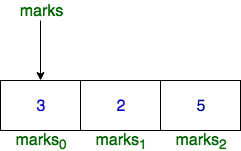## Algorithm

Problem Name: Students Marks Sum

In this HackerRank Functions in C programming problem solution,You are given an array of integers, marks,, denoting the marks scored by students in a class.

• The alternating elements marks0,marks2,marks4 and so on denote the marks of boys.
• Similarly, marks1, marks3, marks0 and so on denote the marks of girls.

The array name, marks, works as a pointer which stores the base address of that array. In other words, marks contains the address where marks0 is stored in the memory.

For example, let marks = |3, 2, 5|and marks stores 0x7fff9575c05f. Then, 0x7fff9575c05f is the memory address of marks0Function Description

Complete the function, marks_summation in the editor below.

marks_summation has the following parameters:

• int marks[number_of_students]: the marks for each student
• int number_of_students: the size of marks[]
• char gender: either 'g' or 'b'

Returns

• int: the sum of marks for boys if gender = b, or of marks of girls if gender = g

Input Format

• The first line contains number_of_students, denoting the number of students in the class, hence the number of elements in marks.
• Each of the number_of_students subsequent lines contains marksi.
• The next line contains gender.

Constraints

• 1 <= number_of_students <= 10***3
• 1 <= marksi <= 10***3 (where 0 <= i <= number_of_students)
• gender = g or b

Sample Input 0

3
3
2
5
b


Sample Output 0

8


## Code Examples

### #1 Code Example with C Programming

Code - C Programming


#include <stdio.h>
#include <string.h>
#include <math.h>
#include <stdlib.h>

//Complete the following function.
int marks_summation(int* marks, int number_of_students, char gender)
{
int sum = 0;

if(gender == 'b'){
for(int i = 0; i < number_of_students; i += 2)
sum += marks[i];
}
else{
for(int i = 1; i < number_of_students; i += 2)
sum += marks[i];
}

return sum;
}

int main()
{
int number_of_students;
char gender;
int sum;

scanf("%d", &number_of_students);
int *marks = (int *) malloc(number_of_students * sizeof (int));

for (int student = 0; student < number_of_students; student++) {
scanf("%d", (marks + student));
}

scanf(" %c", &gender);
sum = marks_summation(marks, number_of_students, gender);
printf("%d", sum);
free(marks);

return 0;
}

Copy The Code &

Input

cmd
3 3 2 5 b

Output

cmd
8